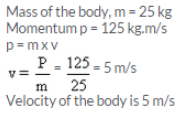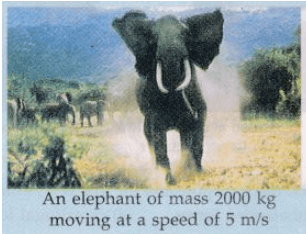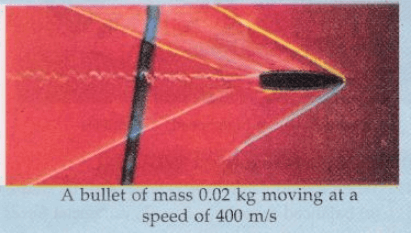Lakhmir Singh & Manjit Kaur: Force and Law of Motion, Solutions- 1

# Lakhmir Singh & Manjit Kaur: Force and Law of Motion, Solutions- 1 - Science Class 9

Page No - 55

Question 1:
What name is given to the product of mass and velocity of a body ?
Solution :
The product of mass and velocity of a body is called momentum.

Question 2:
Name the physical quantity which is considered to be a measure of the quantity of motion of a body.
Solution :
Momentum is the measure of quantity of motion of a body

Question 3:
What is the SI unit of momentum ?
Solution :
The SI unit of momentum is kilogram meters per second(kg.m/s)

Question 4:
State whether momentum is scalar or vector.
Solution :
Momentum is a vector quantity and is dirceted along the direction of velocity.

Question 5:
What is the total momentum of the bullet and the gun before firing ?
Solution :
The total momentum of the bullet and the gun before firing would be zero because velocities of both of them will be zero.

Question 6:
Name the physical quantity whose unit is kg.m/s.
Solution :
Momentum has its SI unit as kilogram meters per second(kg.m/s)

Question 7:
What will be the momentum of a body of mass ‘m’ which is moving with a velocity V ?
Solution :
Momentum of a body of mass ‘m’ and velocity ‘v’ will be
p = m x v

Question 8:
What is the usual name of the forces which cannot produce motion in a body but only change its shape ?
Solution :
Balanced forces cannot produce motion in a body but can its shape.

Question 9:
Name the unbalanced force which slows down a moving bicycle when we stop pedalling it.
Solution :
Frictional force slows down a moving bicycle when we stop pedaling it.

Question 10:
State whether the following statement is true or false :
Unbalanced forces acting on a body change its shape.

Solution :
The given statement is false.

Question 11:
When a ball is dropped from a height, its speed increases gradually. Name the force which causes this change in speed.
Solution :
Force of gravity causes this change in speed.

Question 12:
Name the property of bodies (or objects) to resist a change in their state of rest or of motion.
Solution :
Inertia is the property of bodies to resist a change in their state of rest or motion

Question 13:
What is the other name of Newton’s first law of motion ?
Solution :
Newton’s first law of motion is also known as Galileo’s law of inertia.

Question 14:
The mass of object A is 6 kg whereas that of another object B is 34 kg. Which of the two objects, A or B, has more inertia ?
Solution :
Object B has more inertia. Since mass is a measure of inertia of a body and object B has greater mass, so it will have greater inertia.

Question 15:
Name the scientist who gave the laws of motion.
Solution :
Isaac Newton gave the laws of motion.

Question 16:
State whether force is a scalar or a vector quantity.
Solution :
Force is a vector quantity.

Question 17:
With which physical quantity should the speed of a running bull be multiplied so as to obtain its momentum ?
Solution :
The speed of the running bull should be multiplied with its mass to get its momentum.

Question 18:
Fill in the following blanks with suitable words :
(a) ………………is a measure of the inertia of a body.
(b) When a running car stops suddenly, the passengers are jerked …………………..
(c) When a stationary car starts suddenly, the passengers are jerked …………………..
(d) Newton’s first law of motion is also called Galileo’s law of ………………………
(e) If there were no unbalanced force of……………….. and no…………………. resistance, a moving bicycle would go on moving for ever.

Solution :
a) Mass
b) forward
c) backward
d) inertia
e) friction; air

Question 19:
Explain why, it is easier to stop a tennis ball than a cricket ball moving with the same speed.
Solution :
Since the speed of tennis ball and cricket ball is same, the momentum of cricket ball will be higher due to its mass being greater than mass of tennis ball. So, less force is required to stop a tennis ball than to stop a cricket ball.

Question 20:
Explain the meaning of the following equation : .
p = m x v
where symbols have their usual meanings.

Solution :
p = m x v
This equation signifies that momentum of a body is the product of its mass and its velocity.
Here, p is momentum of the body
m is the mass of the body
v is the velocity of the body.

Question 21:
Explain how, a karate player can break a pile of tiles with a single blow of his hand.
Solution :
A karate player can break a pile of tiles with a single blow because he strikes the pile with his hand very fast. In doing so, the large momentum of his hand is reduced to zero in a very short time. This exerts a large force on the pile of tiles which is sufficient to break them apart.

Question 22:
Calculate the momentum of a toy car of mass 200 g moving with a speed of 5 m/s.
Solution :
Mass of the toy car,
m = 200 g = 0.2 kg
Speed, v = 5 m/s
Momentum, p = m x v
= 0.2 x 5 = 1 kg.m/s

Question 23:
What is the change in momentum of a car weighing 1500 kg when its speed increases from 36 km/h to 72 km/h uniformly ?
Solution :
Mass of car = 1500 kg
Velocity v1 = 36 km/hr = 10 m/s
Momentum p1 = 1500 x 10 = 15000 kg.m/s
Velocity v2 = 72 km/hr = 20 m/s
Momentum p2 = 1500 x 20 = 30000 kg.m/s
Change in momentum = p2-p1= 30000 – 15000 = 15000 kg.m/s

Question 24:
A body of mass 25 kg has a momentum of 125 kg.m/s. Calculate the velocity of the body.
Solution :
Mass of the body, m = 25 kg
Momentum p = 125 kg.m/s
p = m x vQuestion 25:
Calculate the momentum of the following :
(a) an elephant of mass 2000 kg moving at 5 m/s
(b) a bullet of mass 0.02 kg moving at 400 m/sSolution :
a) Mass of elephant = 2000kg
Velocity = 5 m/s
Momentum = 2000 x 5 = 10000 kg.m/s
b) Mass of bullet = 0.02 kg
Velocity = 400 m/s
Momentum = 0.02 x 400 = 8 kg.m/s

The document Lakhmir Singh & Manjit Kaur: Force and Law of Motion, Solutions- 1 | Science Class 9 is a part of the Class 9 Course Science Class 9.
All you need of Class 9 at this link: Class 9

## Science Class 9

66 videos|352 docs|97 tests

## FAQs on Lakhmir Singh & Manjit Kaur: Force and Law of Motion, Solutions- 1 - Science Class 9

 1. What is the force and law of motion?Ans. Force is a physical quantity that can change the state of motion or shape of an object. The law of motion states that an object will remain at rest or in uniform motion unless acted upon by an external force.
 2. What are the three laws of motion?Ans. The three laws of motion, formulated by Sir Isaac Newton, are as follows: 1. First law: An object at rest will remain at rest, and an object in motion will continue to move with a constant velocity unless acted upon by an external force. 2. Second law: The force acting on an object is equal to the mass of the object multiplied by its acceleration. 3. Third law: For every action, there is an equal and opposite reaction.
 3. Can you give an example of the first law of motion?Ans. Yes, an example of the first law of motion is a book placed on a table. The book remains at rest until an external force, such as someone pushing or pulling it, is applied.
 4. How is force calculated according to Newton's second law of motion?Ans. According to Newton's second law of motion, force (F) is calculated by multiplying the mass (m) of an object by its acceleration (a). Mathematically, it can be represented as F = m * a.
 5. What is the significance of Newton's third law of motion?Ans. The significance of Newton's third law of motion is that it explains the concept of action and reaction. It states that for every action, there is an equal and opposite reaction. This law helps us understand how forces are always exerted in pairs and how objects interact with each other.

## Science Class 9

66 videos|352 docs|97 testsExplore Courses for Class 9 examSignup to see your scores go up within 7 days! Learn & Practice with 1000+ FREE Notes, Videos & Tests.
10M+ students study on EduRev
Track your progress, build streaks, highlight & save important lessons and more!
Related Searches

,

,

,

,

,

,

,

,

,

,

,

,

,

,

,

,

,

,

,

,

,

,

,

,

;